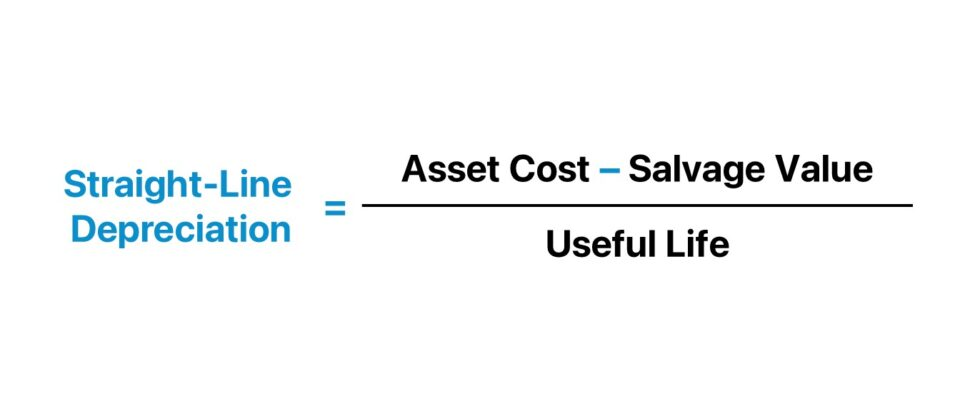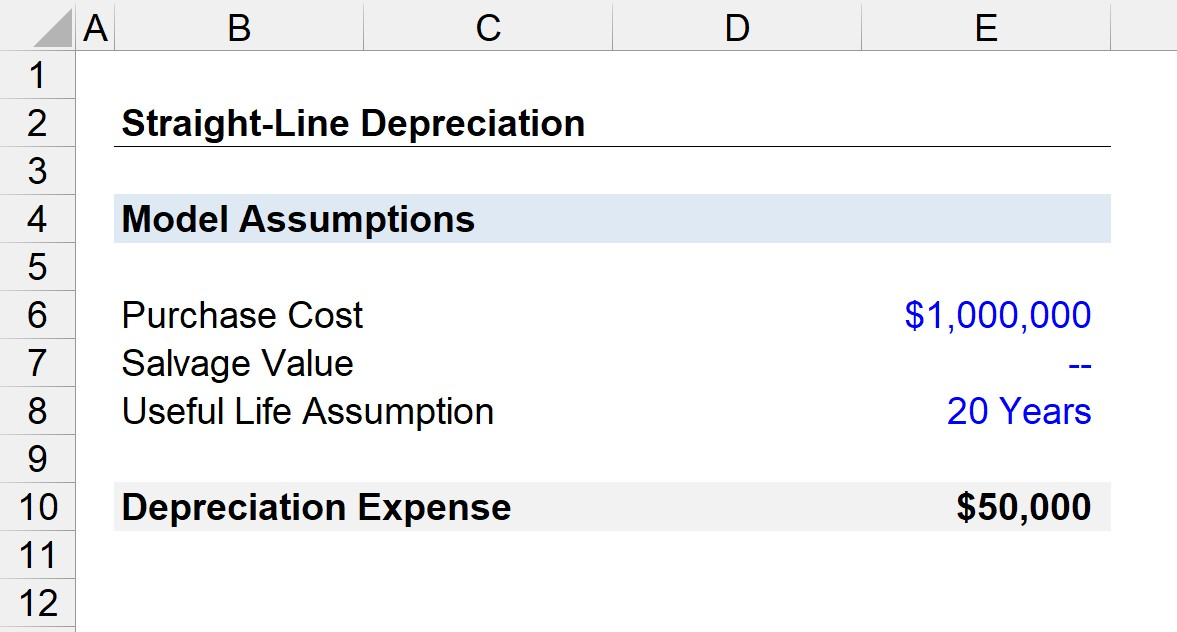Welcome to Wall Street Prep! Use code at checkout for 15% off.# Straight-Line Depreciation

Guide to Understanding Straight-Line Depreciation## How to Calculate Straight-Line Depreciation?

The straight-line depreciation method is characterized by the reduction in the carrying value of a fixed asset recorded on a company’s balance sheet in equal installments.

The straight-line depreciation expense is determined by the following three assumptions:

1. Asset Cost → The initial cost of purchasing the fixed asset, or the capital expenditure (Capex).
2. Salvage Value (“Residual Value”): The residual value of the fixed asset at the end of its useful life.
3. Useful Life → The number of years in which a fixed asset is expected to continue contributing positive economic benefits.

Taking a step back, the concept of depreciation in accounting stems from the purchase of fixed assets (PP&E) via capital expenditures (Capex).

Unlike current assets such as inventory, PP&E is expected to offer positive economic value for more than one-year (>12 months).

Further, depreciation can be thought of as the gradual decline in value of a fixed asset (PP&E) over its useful life assumption, which is the estimated duration in which a non-current fixed asset is expected to provide economic benefits.

Under the matching principle in accrual accounting, the costs associated with an asset with long-term benefits must be recognized in the same period for consistency.

Hence, the depreciation line item – which is typically embedded within either cost of goods sold (COGS) or operating expenses (OpEx) – is a non-cash expense, as the real cash outflow occurred earlier when the Capex was spent.

There are a couple of accounting approaches for calculating depreciation, but the most common one is straight-line depreciation.

## Straight-Line Depreciation Formula

In the straight line method of depreciation, the value of an asset is reduced in equal installments in each period until the end of its useful life.

The formula consists of dividing the difference between the initial capital expenditure (Capex) amount and the anticipated salvage value at the end of its useful life by the total useful life assumption.

Straight-Line Depreciation = (Purchase Price Salvage Value) ÷ Useful Life

Where:

• Purchase Price → The total cost incurred to purchase the fixed asset (PP&E)
• Salvage Value → The estimated value of the fixed asset at the end of its useful life. Usually, the salvage value (i.e. the residual value that that asset could be sold for) at the end of the asset’s useful life is assumed to be zero.
• Useful Life → The number of years that the fixed asset (PP&E) is expected to continue providing positive economic value to the company.

## Straight-Line Depreciation Calculator

We’ll now move to a modeling exercise, which you can access by filling out the form below.#### Get the Excel Template!Submitting...

## 1. Fixed Asset and Capex Assumptions

Suppose a hypothetical company recently incurred \$1 million in capital expenditures (Capex) to purchase fixed assets.

Per guidance from management, the fixed assets have a useful life of 20 years, with an estimated salvage value of zero at the end of their useful life period.

• Purchase Cost = \$1 million
• Useful Life = 20 Years
• Salvage Value = \$0

## 2. Straight-Line Depreciation Calculation Example

In the next section, we’ll start by calculating the numerator, the purchase cost subtracted by the salvage value.

But since the salvage value is zero, the numerator is equivalent to the purchase cost (i.e. \$1 million).

After dividing the \$1 million purchase cost by the 20-year useful life assumption, we arrive at \$50k for the annual depreciation expense.

• Straight-Line Depreciation = \$1 million ÷ 20 years = \$50kStep-by-Step Online Course

### Everything You Need To Master Financial Modeling

Enroll in The Premium Package: Learn Financial Statement Modeling, DCF, M&A, LBO and Comps. The same training program used at top investment banks.